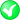#Boost C++ Libraries

...one of the most highly regarded and expertly designed C++ library projects in the world.

##### within (with strategy)

Checks if the first geometry is completely inside the second geometry using the specified strategy.

###### Description

The free function within checks if the first geometry is completely inside the second geometry, using the specified strategy. Reasons to specify a strategy include: use another coordinate system for calculations; construct the strategy beforehand (e.g. with the radius of the Earth); select a strategy when there are more than one available for a calculation.

###### Synopsis

```template<typename Geometry1, typename Geometry2, typename Strategy>
bool within(Geometry1 const & geometry1, Geometry2 const & geometry2, Strategy const & strategy)```

###### Parameters

Type

Concept

Name

Description

Geometry1 const &

Any type fulfilling a Geometry Concept

geometry1

A model of the specified concept which might be within the second geometry

Geometry2 const &

Any type fulfilling a Geometry Concept

geometry2

A model of the specified concept which might contain the first geometry

Strategy const &

strategy

strategy to be used

###### Returns

true if geometry1 is completely contained within geometry2, else false

Either

`#include <boost/geometry/geometry.hpp>`

Or

`#include <boost/geometry/algorithms/within.hpp>`

###### Conformance

The function within implements function Within from the OGC Simple Feature Specification.

NoteOGC defines within as completely within and not on the border. See the notes for within / on the border
###### Supported geometries

Point

Segment

Box

Linestring

Ring

Polygon

MultiPoint

MultiLinestring

MultiPolygon

PointSegmentBoxLinestringRingPolygonMultiPointMultiLinestringMultiPolygonNoteIn this status matrix above: columns are types of first parameter and rows are types of second parameter. So a point can be checked to be within a polygon, but not vice versa.
###### Notes

If a point is located exactly on the border of a geometry, the result depends on the strategy. The default strategy (Winding (coordinate system agnostic)) returns false in that case.

If a polygon has a reverse oriented (e.g. counterclockwise for a clockwise typed polygon), the result also depends on the strategy. The default strategy returns still true if a point is completely within the reversed polygon. There is a specific strategy which returns false in this case.

Linear